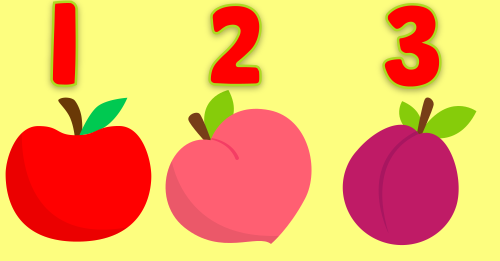Let's do some simple calculations.Can you tell the first letters of these words?How many helicopters are there?Can you tell the order of these animals?Can you count these watermelons?Let's do some simple calculations.Can you count these pears?Let's count giraffes together!Do you know the first letters of these words?Which one is the animal that we want?Can you count these tomatoes and eggplants?Let's do some simple calculations.Can you tell how many excavators are there?Let's count chickens together!Can you tell the first letters of these words of foods?Can you tell the first letters of these words of vegetables?Can you find the missing letters?Can you count these onions and garlics?Which vegetable is bigger?